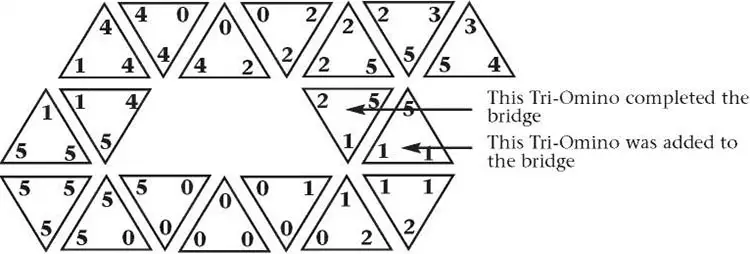• #### Other Games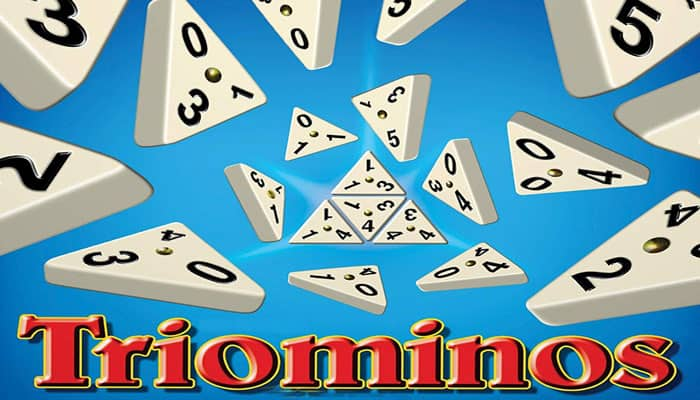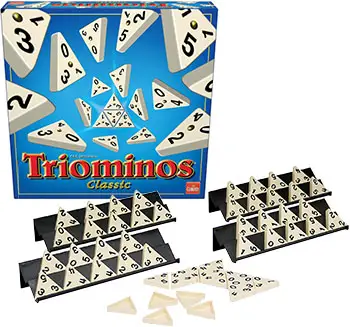## Components

• 56 Tri-Omino tiles
• 4 Racks.
• Rulebook

## Object of the Game

Be the first player to score 400 points. Each game consists of several rounds.

## Setup

Place the Tri-Ominos face down and mix them. On a piece of paper, write down the name of each player. As each play is made, the scorekeeper will add or subtract points in the proper column.

Each player takes the required number of Tri-Ominos as follows:

• 2 players 9 each
• 3 to 4 players 7 each

Players place the tiles on the rack with the numbers facing them. Do not let the other players see your tiles.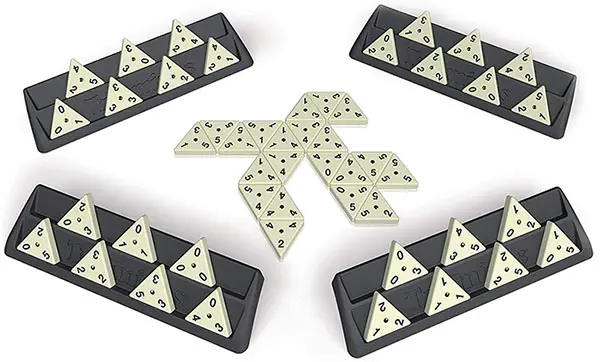## Game Play

The player that has the Tri-Omino with three of the same highest numbers starts the round by placing the Tri-Omino face up on the table.

Three 5's is the highest, then three 4's, etc. The starting player receives the total of the three numbers on the Tri-Omino plus a 10 point bonus. If three Os start, there is a 30 point bonus, plus the normal 10 point bonus.

Example: 4 x 3 = 12 + 10 = 22 points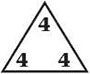The player that has the starting Tri-Omino and the three Os has the option of playing either one. If this player chooses to start with the three 0s to score the additional bonus points, he must show the other play- ers the Tri-Omino with the same highest number on it.

If no player has a Tri-Omino with three of the same number on it, the player with the highest total on one Tri-Omino starts. This player scores the total of the numbers on that Tri-Omino, but does not score any bonus points.

Example: 5 + 4 + 3 = 12 points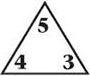In clockwise order, the next player tries to match any two numbers on the starting player's Tri-Omino with one of his Tri-Ominos. If the player can do this, he scores the total of the three numbers on his Tri-Omino.

Example: 3 + 4 + 4 = 11 points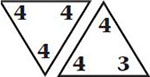5 + 5 + 2 = 12 points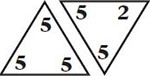## Picking from the Well

If you can't make a match, pick from the "well" (remaining Tri-Ominos that are face down). Keep these Tri-Ominos separate from the other Tri-Ominos in your hand until you pick a match.

For each Tri-Omino picked from the well, the scorekeeper deducts 5 points from your score. After the deduction is made, the scorekeeper adds the total sum of points from the matching Tri-Omino to your score.

If you can't match any Tri-Omino in your hand and there are none in the well, ten points are deducted from your score and play continues.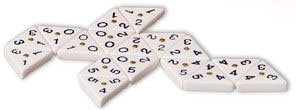## Winning a Round

The first player to play all of his Tri-Ominos wins the round and receives a 25 point bonus plus the total points of all Tri-Ominos left in the other players' hands. The other players do not deduct these points from their score.

If all players have passed, the game is blocked and the player with the least amount of Tri-Ominos wins the round. A

ll points from the other players' hands are added to this players' score, but there are no bonus points. This player must also deduct the total number of points that were left in his hand from his score.

To begin a new round, turn all the Tri-Ominos face down, mix them and each player picks new ones. Every round is played the same way as the first, beginning with the player who now has the Tri-Omino with the same highest number on it.

## End of the Game

The first player to reach 400 points wins the game. If a player reaches 400 points in the middle of a round, continue playing until the round is over.

If more than one player passes 400 points during the round, the winner of that round wins the game.

#### Bonus Scoring:

If a player matches all three numbers of a Tri-Omino and forms a closed hexagon, add the sum of the three numbers plus a 50 point bonus to his score.

Example: 0 + 5 + 5 = 10 + 50 = 60 points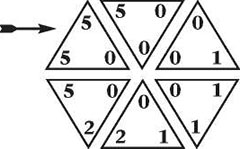If a player matches a Tri-Omino and forms a bridge, add the sum of the three numbers plus a 40 point bonus to his score. A bridge is formed by matching one side of a Tri-Omino and the point opposite.

Example:: 1 + 4 + 4 = 9 + 40 = 49 points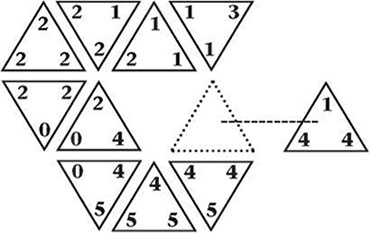After a bridge has been formed and a player matches two sides of a Tri-Omino, he receives the sum of the three numbers plus a 40 point bonus.

Example: 1 + 1 + 5 = 7 + 40 = 47 points